# Week 2: Image and Video Upscaling from Local Self-Examples

Definitions

• Dyadic Filters: Filters that implement that use octave bands.
• Octave band filters: These filters separate the complete frequency spectrum into a set of frequencies.
• Octave band: An octave band is defined when upper band frequency is twice the lower band frequency.
• 1/3 Octave band filter: Defined when upper band frequency is the lower and freq times cube root of two ($f_{high}=f_{low}\sqrt{2}$)

This post is based on the paper written by GILAD FREEDMAN and RAANAN FATTAL at the Hebrew University of Jerusalem.  I will be trying to analyze and provide an overview and hopefully provide a complete understanding about how their image upscaling algorithm works.

Concept

Freedman and Fattal’s upscaling algorithm is based on the idea that in a certain group of pixels, other nearby pixels will present a similarity.  Using this idea, their algorithm will be able gather example data and produce an educated interpolation based upon this.  Hence the title of the paper “Local Self Examples”.  They contrasted their algorithm with global searches and database searches and showed that their algorithm is almost an order faster than many others because there is no need to expand the search to a significantly larger search radius.

It is relevant to note that due to the small size of the search area, using large upscaling factors will be detrimental and produce undesired results.  In order to scale to a larger factor one must run the algorithm multiple times.

In addition to this they are using the YCbCr color scheme as it has proven to be faster than the RGB color scheme.  This is because they only had to modify the Y component instead of all three of the RGB components.

Local Self-Similarity

The idea here is that the source image has the most similarities in it because that is the picture you’re trying to process.  Thus compared to external databases with high resolution images (but not your exact image) it is easy to see why similarities are much more prevalent.

That begs the question what similarities are we using to predict for interpolation?  One of the largest problems with image upscaling is retaining the sharpness of edges in images .

Thus it would only be natural to use these edges of the source image as patches of “similarity”.  In addition because they come from the same source image, Freedman and Fattal call this local self-similarity.

However, these patches are only several pixels wide.  Quoting their experimental results, using patches of 5×5 pixels with search area 10×10 pixels (Local) and 20×20 pixels (Local 2) they show that on scale with error versus time required to process (noting small scaling factor of 5(upscaled):4(source)) that their algorithm is much superior.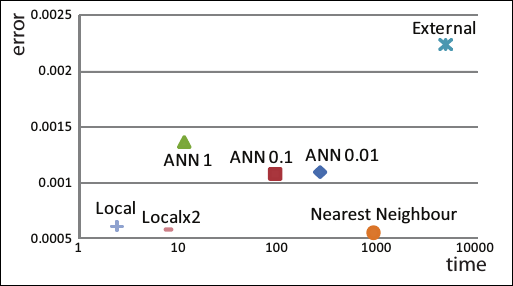Fig 9 from Freedman and Fattal: External=External database sources, Nearest Neighbor: linear interpolation, ANN: Approximate Nearest Neighbor: linear interpolation

Algorithm

The algorithm can be defined in the following steps. Given input image$I_{0}$. Operators for upsampling and downsampling are given using the non-dyadic filters design by Freedman and Fattal.

1. Use interpolation factor U to create a finer grid of pixels from grid$G_{l}$ to$G_{l+1}$
•$L_{1}=U(I_{0})$
2.  Because$L_{1}$ is lacking high frequency components, due to attenuation, of the source image obtain high frequency components from the source image to produce$L_{0}$ (low freq content smoothed image) where D is defined as the downsampling operator which maps$G_{l}$ to$G_{l-1}$
•$L_{0}=U(D(I_{0}))$
3. Compare local patches$p$ (small radius) of$G_{1}$ to$G_{0}$ the most similar patch in$G_{0}$ defined as$q$
4. Compute the lacking high frequency content in$L_{1}$ by doing the following, where$q$ represent the low frequency locations in the source image
•$H_{0}=I_{0}(q)-L_{0}(q)$
5. Add the high frequency image to the interpolated$L_{1}$ and compute final output image
•$I_{p}=L_{1}(p)+H_{0}(q(p))$

Conditions for using Freedman and Fattal’s algorithm

1. Uniform Scaling: Every pixel mapped from an upscale or downsample to a new image must be the same distance from each other
•$L_{0}(0,0)$->$L_{1}(0,0)$,$L_{0}(0,1)$->$L_{1}(0,5)$,$L_{0}(0,2)$->$L_{1}(0,10)$
2. Low Frequency Span: Images taken from cameras have been low pass filtered to prevent aliasing of the higher frequencies, thus both U and D need to have low pass properties.
3. Singularities Preservation: Singularities, as defined above, must be retained after performing an operation.  This means that$L_{0}=U(D(I_{0}))$ and$L_{1}=U(I_{0})$ must have similar edge properties after upscaling.  This also means that$I_{-1}$ produced from downsampling must also retain these edges.
4. Consistent and Optimal Reproduction: The D and U operators must be invertible meaning the filters must be biorthogonal.

Freedman and Fattal’s Non-Dyadic Upscaling and Downscaling Equations

Dyadic filters as explained here in terms of image upscaling will upscale by 2.  Thus when it comes to Freedman and Fattal’s filters which use a modified wavelet transform we see scaling factors other than two.

Freedman and Fattal use a N+1:N scaling ratio (5 pixels in upscaled 4 pixels in source).  This works under the assumption that within a N+1 period of pixels, the picture is spatially invariant.  Otherwise shifting over a N+1 period we can expect variance in the pixels.

Knowing this Freedman and Fattal define their upsampling and downsampling schemes as follows.$D(I)(n)=(I\ast \bar{d_{p}})((N+1)q+p)$ Where D represents the downsampled results, I represents the image, n represents the index of a pixel, the * represents convolution$\bar{d_{p}}=d[-n]$ represents the filter coefficients, p=n mod N (for the N+1 image$G_{l+1})$ , q=(n-p)/N (for the$G_{l}$)$U(I)(n)=\sum_{k=1}^{N}(\uparrow I*\bar{u_{k}})(n)$ Where U represents the upsampled results, I represents the input image, n represents the index of the pixel, the * represents convolution$\bar{u_{k}}=u[-k]$ represents the filter coefficients.

Under the assumptions above, we can assume that these are biorthogonal filters which means they are invertible.   Ensuring that$I=D(U(I))$ is valid.

Filter Derivation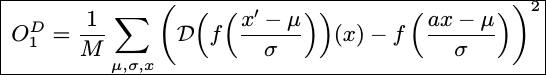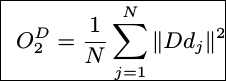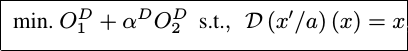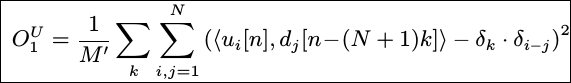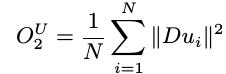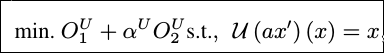I will update my understanding of this later.  For now the gist of the idea is that.  The nonlinear relation created by the biorthogonality condition is split into two linear portions in order to lower the complexity of the filter.  Part 1 satisfies the third condition of singularity preservation and the second part is to minimize power through Parsevel’s theorem.  The alpha term is to choose to prioritize either satisfying singularity or power minimization.  We choose whichever is the largest to minimize.

Source:

Freedman, Gilad, and Raanan Fattal. “Image and Video Upscaling from Local Self-Examples.” Image and Video Upscaling from Local Self-Examples(2009): n. pag. Print. http://doi.acm.org/10.1145/1559755.1559763